## FCSys.Species

Dynamic models of chemical species

### Information

Extends from Modelica.Icons.Package (Icon for standard packages).

### Package Content

NameDescription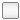'C+' C'SO3-' C19HF37O5S- (abbreviated as SO3-)'e-' e-'H+' H+H2 H2H2O H2ON2 N2O2 O2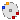Ion Base model for an ionFluid Base model for a fluid species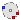Solid Base model for an inert, stationary solid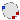Species Base model for one chemical species in one phase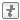Enumerations Choices of options

##FCSys.Species.Ion

Base model for an ion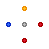### Information

Extends from Fluid (Base model for a fluid species).

### Parameters

TypeNameDefaultDescription
Integern_inter0Number of exchange connections with other phases
Material properties
replaceable package DataCharacteristics.BaseClasses….Characteristic data
Mobilitymusigma*vMobility [N.T/M]
TimeAbsolutenuData.nu(T, v)Thermal independity [T]
Independence factors
NumberAbsolutek_intra_Phi[n_intra, n_trans]ones(n_intra, n_trans)For translational exchange among species within the phase 
NumberAbsolutek_intra_Q[n_intra]ones(n_intra)For thermal exchange among species within the phase 
Initialization
Velocityphi.start[n_trans]0Velocity [L/T]
CurrentI.start[n_trans]0Current [N/T]
Velocityphi_boundaries.start[n_trans, Side]0Normal velocities at the boundaries [L/T]
Forcef.start[n_trans]0Total normal translational force on pairs of boundaries [L.M/T2]
ForceminusDeltaf.start[n_trans]0Dynamic and nonequilibrium compression forces [L.M/T2]
Chemical parameters
TimeAbsolutetauprime[n_chem]zeros(n_chem)Specific exchange currents [T]
Geometry
LengthkL[:]L[cartTrans]Effective transport length [L]
Initialization
InitinitMaterialInit.pressureMethod of initializing the material state
InitinitEnergyInit.temperatureMethod of initializing the thermal state
AmountN_IC Initial amount of material [N]
Densityrho_IC Initial density [N/L3]
VolumeV_IC Initial volume [L3]
PressureAbsolutep_IC Initial pressure [M/(L.T2)]
TemperatureAbsoluteT_IC Initial temperature [L2.M/(N.T2)]
Potentialh_IC Initial specific enthalpy [L2.M/(N.T2)]
Potentialg_IC Initial Gibbs potential [L2.M/(N.T2)]
Assumptions
Integern_trans1Number of transport axes
Integern_chem0Number of reaction and phase change processes
Formulation of the conservation equations
ConsThermoconsMaterialConsThermo.dynamicMaterial
BooleanconsRotfalseConserve rotational momentum
ConsTransconsTransXConsTrans.dynamicX-axis translational momentum
ConsTransconsTransYConsTrans.dynamicY-axis translational momentum
ConsTransconsTransZConsTrans.dynamicZ-axis translational momentum
ConsThermoconsEnergyConsThermo.dynamicEnergy
Axes with upstream discretization
BooleanupstreamXtrueX
BooleanupstreamYtrueY
BooleanupstreamZtrueZ
Flow conditions
BooleanapproxVelocitytrueCalculate normal boundary velocities assuming uniform density
NumberAbsoluteNu_Phi[Axis]{4,4,4}Translational Nusselt numbers 
NumberAbsoluteNu_Q1Thermal Nusselt number 
AmountN00Nominal amount of material to prevent depletion [N]

### Connectors

TypeNameDescription
Intraintra[n_intra]Connectors to exchange translational momentum and energy within the phase
Interinter[n_inter]Connectors to exchange translational momentum and energy with all other species
Boundaryboundaries[n_trans, Side]Connectors for transport
Chemicalchemical[n_chem]Connector for reactions and phase change

### Modelica definition

```model Ion "Base model for an ion"
import assert = FCSys.Utilities.assertEval;
extends Fluid(final mu=sigma*v, N(stateSelect=StateSelect.default));

parameter Q.ConductivityElectrical sigma=Data.mu()/Data.v_Tp()
"Electrical conductivity";

end Ion;
```

##FCSys.Species.Fluid

Base model for a fluid species### Information

Fixed assumptions:

1. The gradient of material current is uniform in the direction of the current.
2. The normal translational force on pairs of boundaries is split equally between the boundaries. This includes the body, shear (transverse translational transport), and exchange forces due to intermolecular drag and transfer during chemical reactions and phase change. It excludes the thermodynamic, dynamic (advective normal translational transport), and nonequilibrium (irreversible compression) pressures. It also excludes transient effects since translational momentum is stored at the boundaries (not in the subregion).
3. Nonequilibrium pressure is included in the thermodynamic states at the boundaries. In particular, the specific enthalpy at a boundary is a function of the temperature and the sum of the thermodynamic and nonequilibrium pressures at the boundary (and a possible artifact of dynamic pressure; see the first note regarding parameters). The rate of advection of energy is the product of this specific enthalpy and the material current.

Notes regarding the parameters:

1. If `approxVelocity` is `true`, then the normal velocities at the boundaries are calculated from the boundary currents assuming that the density is uniform. This avoids nonlinear systems of equations, but it introduces an artifact of the dynamic pressure into the thermodynamic states at the boundaries. The extra pressure is m i2 (v - vi)/A′, where m is the specific mass, v is the specific volume in the subregion, vi is the specific volume at the boundary, i is the boundary current, and A′ is the available cross-sectional area. This affects the energy balance via the specific enthalpy at the boundaries.
2. If `consTransX`, `consTransY`, or `consTransZ` is `ConsTrans.steady`, then the derivative of translational momentum at and normal to the boundaries (proportional to ∂i/∂t) is treated as zero and removed from the translational momentum balances/material transport equations at the corresponding boundaries.
3. If consRot is `true`, then rotational momentum is conserved without storage (i.e., steady). This means that the shear forces are mapped so that there is no net torque around any rotational axis that has all its boundaries included (i.e., all the boundaries around the perimeter). Rotational momentum is not exchanged among species or directly transported (i.e., uniform or shaft rotation).
4. Upstream discretization is applied by default. The central difference scheme may be used by setting `upstreamX`, `upstreamY`, and `upstreamZ` to `true`. The typical diffusion properties are such that the Péclet number for the upstream discretization of pressure will be much less (factor of 1/10,000) than the Péclet numbers for translational and thermal transport. Therefore, it may appear that pressure is not advected with the material transport stream.
5. The indices of the translational Nusselt number (NuΦ) correspond to the orientation of the translational momentum that is transported, not the axes of material transport.
6. The default thermal Nusselt number is one, which represents pure conduction through the gas. Use 3.66 for internal flow where the boundaries are uniform in temperature or 48/11 (approximately 4.36) if the heat flux is uniform [Incropera2002].

Translational momentum and thermal energy are advected as material is exchanged due to phase change and reactions. This occurs at the velocity (φ) and the specific entropy-temperature product (sT) of the reactants (source configurations), where the reactant/product designation depends on the current conditions.

The advective exchange is modeled via a `stream` connector (Chemical). The rate of advection of translational momentum is the product of the velocity of the source (φ) and the mass flow rate ( or m). The rate of thermal advection is the specific entropy-temperature product of the source (sT) times the rate of material exchange (). If there are multiple sources, then their contributions are additive. If there are multiple sinks, then translational momentum is split on a mass basis and the thermal stream is split on a particle-number basis.

Extends from Species (Base model for one chemical species in one phase).

### Parameters

TypeNameDefaultDescription
Integern_inter0Number of exchange connections with other phases
Material properties
replaceable package DataCharacteristics.BaseClasses….Characteristic data
MobilitymuData.mu(T, v)Mobility [N.T/M]
TimeAbsolutenuData.nu(T, v)Thermal independity [T]
Independence factors
NumberAbsolutek_intra_Phi[n_intra, n_trans]ones(n_intra, n_trans)For translational exchange among species within the phase 
NumberAbsolutek_intra_Q[n_intra]ones(n_intra)For thermal exchange among species within the phase 
Initialization
TemperatureAbsoluteT.startT_ICTemperature [L2.M/(N.T2)]
Velocityphi.start[n_trans]0Velocity [L/T]
Chemical parameters
TimeAbsolutetauprime[n_chem]zeros(n_chem)Specific exchange currents [T]
Geometry
LengthkL[:]L[cartTrans]Effective transport length [L]
Initialization
InitinitMaterialInit.pressureMethod of initializing the material state
InitinitEnergyInit.temperatureMethod of initializing the thermal state
AmountN_IC Initial amount of material [N]
Densityrho_IC Initial density [N/L3]
VolumeV_IC Initial volume [L3]
PressureAbsolutep_IC Initial pressure [M/(L.T2)]
TemperatureAbsoluteT_IC Initial temperature [L2.M/(N.T2)]
Potentialh_IC Initial specific enthalpy [L2.M/(N.T2)]
Potentialg_IC Initial Gibbs potential [L2.M/(N.T2)]
Assumptions
Integern_trans1Number of transport axes
Integern_chem0Number of reaction and phase change processes
Formulation of the conservation equations
ConsThermoconsMaterialConsThermo.dynamicMaterial
BooleanconsRotfalseConserve rotational momentum
ConsTransconsTransXConsTrans.dynamicX-axis translational momentum
ConsTransconsTransYConsTrans.dynamicY-axis translational momentum
ConsTransconsTransZConsTrans.dynamicZ-axis translational momentum
ConsThermoconsEnergyConsThermo.dynamicEnergy
Axes with upstream discretization
BooleanupstreamXtrueX
BooleanupstreamYtrueY
BooleanupstreamZtrueZ
Flow conditions
BooleanapproxVelocitytrueCalculate normal boundary velocities assuming uniform density
NumberAbsoluteNu_Phi[Axis]{4,4,4}Translational Nusselt numbers 
NumberAbsoluteNu_Q1Thermal Nusselt number 
AmountN00Nominal amount of material to prevent depletion [N]

### Connectors

TypeNameDescription
Intraintra[n_intra]Connectors to exchange translational momentum and energy within the phase
Interinter[n_inter]Connectors to exchange translational momentum and energy with all other species
Boundaryboundaries[n_trans, Side]Connectors for transport
Chemicalchemical[n_chem]Connector for reactions and phase change

### Modelica definition

```model Fluid "Base model for a fluid species"

import FCSys.Utilities.Coordinates.after;
import FCSys.Utilities.Coordinates.before;
import FCSys.Utilities.Coordinates.cartWrap;
import FCSys.Utilities.Delta;
import FCSys.Utilities.Sigma;
import FCSys.Utilities.inSign;
import FCSys.Utilities.selectBooleans;
import FCSys.Utilities.selectIntegers;
import assert = FCSys.Utilities.assertEval;

// Initialization parameters
parameter Init initMaterial=Init.pressure
"Method of initializing the material state";
parameter Init initEnergy=Init.temperature
"Method of initializing the thermal state";
// Note:  The extension is after these parameters so that they appear first
// in the parameter dialog.
extends Species(N(stateSelect=if consMaterial == ConsThermo.dynamic then
StateSelect.always else StateSelect.prefer),T(final fixed=false));
// Note:  StateSelect.always isn't ideal, but it's necessary to avoid
// dynamic state selection in Dymola 2014.  In some cases it isn't
// appropriate (e.g., an incompressible liquid that fills the entire
// subregion), and in those cases it can be modified at instantiation.

// Material properties
parameter Integer n_chem=0 "Number of reaction and phase change processes";
Q.DiffusivityMassSpecific zeta(nominal=1e-3*U.N/U.A) = Data.zeta(T, v) "**";
Q.Fluidity eta(nominal=1e5/(U.Pa*U.s)) = Data.eta(T, v) "Fluidity";
Q.ResistivityThermal theta(nominal=10*U.m*U.K/U.W) = Data.theta(T, v)
"Thermal resistivity";

// Chemical parameters
Q.TimeAbsolute tauprime[n_chem](each nominal=U.ms) = zeros(n_chem)
"Specific exchange currents";

// Geometry
Q.Length kL[:]=L[cartTrans] "Effective transport length";
// Note:  The size is n_trans, but it isn't specified here to
// prevent a warning in Dymola 2014.

// Assumptions
// -----------
// Dynamics
parameter ConsThermo consMaterial=ConsThermo.dynamic "Material";
parameter Boolean consRot=false "Conserve rotational momentum";

parameter ConsTrans consTransX=ConsTrans.dynamic
"X-axis translational momentum";
parameter ConsTrans consTransY=ConsTrans.dynamic
"Y-axis translational momentum";
parameter ConsTrans consTransZ=ConsTrans.dynamic
"Z-axis translational momentum";
parameter ConsThermo consEnergy=ConsThermo.dynamic "Energy";
//
// Upstream discretization
parameter Boolean upstreamX=true "X";
parameter Boolean upstreamY=true "Y";
parameter Boolean upstreamZ=true "Z";
//
// Flow conditions
parameter Boolean approxVelocity=true
"Calculate normal boundary velocities assuming uniform density";
parameter Q.NumberAbsolute Nu_Phi[Axis]={4,4,4}
"Translational Nusselt numbers";
parameter Q.NumberAbsolute Nu_Q=1 "Thermal Nusselt number";

parameter Q.Amount N0=0 "Nominal amount of material to prevent depletion";

// Aliases (for common terms)
Q.Current I[n_trans](
each nominal=U.A,
each stateSelect=StateSelect.never,
each start=0) "Current";
Q.Velocity phi_boundaries[n_trans, Side](
each nominal=100*U.cm/U.s,
each stateSelect=StateSelect.never,
each start=0) "Normal velocities at the boundaries";
Q.Force f[n_trans](
each nominal=U.N,
each stateSelect=StateSelect.never,
each start=0) "Total normal translational force on pairs of boundaries";
// This (f) includes the body, shear, and exchange forces due to
// intermolecular drag and transfer during chemical reactions and phase
// change.  It excludes the thermodynamic, dynamic, and  nonequilibrium
// compressive forces.  It also excludes transient effects since
// translational momentum is stored at the boundaries.
Q.Force minusDeltaf[n_trans](
each nominal=U.N,
each stateSelect=StateSelect.never,
each start=0) "Dynamic and nonequilibrium compression forces";

// Auxiliary variables (for analysis)
// ----------------------------------
// Misc. conditions
output Q.Density rho_boundaries[n_trans, Side](each stateSelect=StateSelect.never)
= fill(
1,
n_trans,
2) ./ Data.v_Tp(boundaries.T, boundaries.p) if environment.analysis
"Densities at the boundaries";
output Q.VolumeRate Vdot_boundaries[n_trans, Side](each stateSelect=
StateSelect.never) = boundaries.Ndot ./ rho_boundaries if environment.analysis
"Volume flow rates into the boundaries";
output Q.PressureAbsolute q[n_trans](each stateSelect=StateSelect.never) = (
Data.m/2)*phi .* I ./ Aprime if environment.analysis "Dynamic pressure";
output Q.Velocity phi_chemical[n_chem, n_trans](each stateSelect=StateSelect.never)
= actualStream(chemical.phi) if environment.analysis and n_chem > 0
"Velocity of the chemical streams";
output Q.PotentialAbsolute sT_chemical[n_chem](each stateSelect=StateSelect.never)
= actualStream(chemical.sT) if environment.analysis and n_chem > 0
"Specific entropy-temperature product of the chemical streams";
output Q.Temperature DeltaT[n_trans](each stateSelect=StateSelect.never) =
Delta(boundaries.T) if environment.analysis
"Differences in temperatures across the boundaries";
output Q.Pressure Deltap[n_trans](each stateSelect=StateSelect.never) = Delta
(boundaries.p) if environment.analysis
"Differences in pressures across the boundaries";
output Q.Power Hprimedot[n_trans, Side](each stateSelect=StateSelect.never)
= (Data.h(boundaries.T, boundaries.p) + Data.m*phi_boundaries .^ 2/2) .*
boundaries.Ndot if environment.analysis
"Flow rates of enthalpy + kinetic energy into the boundaries";
//
// Potentials
output Q.Potential g_boundaries[n_trans, Side](each stateSelect=StateSelect.never)
= Data.g(boundaries.T, boundaries.p) if environment.analysis and not Data.isCompressible
"Gibbs potentials at the boundaries";
output Q.Potential Deltag[n_trans](each stateSelect=StateSelect.never) =
Delta(g_boundaries) if environment.analysis and not Data.isCompressible
"Differences in Gibbs potentials across the boundaries";
// Note:  If a boundary is left unconnnected, then it's possible that its
// pressure may become negative.  If the equation of state has an ideal-gas
// term, then the Gibbs energy will involve a logarithm of pressure.
// Therefore, to prevent errors, these variables are included only for
// incompressible species.
//
// Time constants
output Q.TimeAbsolute tau_NT[n_trans](
each stateSelect=StateSelect.never,
each start=U.s) = fill(zeta*beta*N, n_trans) ./ (2*Aprime) if environment.analysis
"Time constants for material transport";
output Q.TimeAbsolute tau_PhiT[n_trans](
each stateSelect=StateSelect.never,
each start=U.s) = M*eta*kL ./ (2*Nu_Phi[cartTrans] .* Aprime) if
environment.analysis
"Time constants for transverse translational transport";
output Q.TimeAbsolute tau_QT[n_trans](
each stateSelect=StateSelect.never,
each start=U.s) = (N*c_v*theta/(2*Nu_Q))*kL ./ Aprime if environment.analysis
"Time constants for thermal transport";
//
// Peclet numbers
output Q.Number Pe_N[n_trans](each stateSelect=StateSelect.never) = tau_NT .*
I/N if environment.analysis "Material Peclet numbers";
output Q.Number Pe_Phi[n_trans](each stateSelect=StateSelect.never) =
tau_PhiT .* I/N if environment.analysis "Translational Peclet numbers";
output Q.Number Pe_Q[n_trans](each stateSelect=StateSelect.never) = tau_QT .*
I/N if environment.analysis "Thermal Peclet numbers";
// Note:  These Peclet numbers are calculated at the center of the
// subregion (for simplicity), but the Peclet numbers used in the transport
// equations are at each boundary.
//
// Bulk flow rates
output Q.Force mphiI[n_trans, n_trans](each stateSelect=StateSelect.never) =
outerProduct(I, Data.m*phi) if environment.analysis
"Bulk rate of translational advection (1st index: transport axis, 2nd index: translational component)";
output Q.VolumeRate Vdot[n_trans](each stateSelect=StateSelect.never) = v*I
if environment.analysis "Bulk volumetric flow rate";
output Q.Power hI[n_trans](each stateSelect=StateSelect.never) = h*I if
environment.analysis "Bulk enthalpy flow rate";
//
// Translational momentum balance
output Q.Force Ma[n_trans](each stateSelect=StateSelect.never) = M*(der(phi)/
U.s + environment.a[cartTrans]) + N*Data.z*environment.E[cartTrans] if
environment.analysis
"Acceleration force (including acceleration due to body forces)";
output Q.Force f_thermo[n_trans](each stateSelect=StateSelect.never) = -Delta
(boundaries.p) .* Aprime if environment.analysis "Thermodynamic force";
output Q.Force f_AE[n_trans](each stateSelect=StateSelect.never) = Data.m*sum
((actualStream(chemical[i].phi) - phi)*chemical[i].Ndot for i in 1:n_chem)
if environment.analysis "Acceleration force due to advective exchange";
output Q.Force f_AT[n_trans](each stateSelect=StateSelect.never) = {sum(((if
i == j then phi_boundaries[j, :] else boundaries[j, :].phi[cartWrap(
cartTrans[i] - cartTrans[j])]) - {phi[i],phi[i]})*boundaries[j, :].Ndot*
Data.m for j in 1:n_trans) for i in 1:n_trans} if environment.analysis
"Acceleration force due to advective transport";
output Q.Force f_DT[n_trans](each stateSelect=StateSelect.never) = {sum(sum(
if i == j then {0,0} else boundaries[j, :].mPhidot[cartWrap(cartTrans[i] -
cartTrans[j])]) for j in 1:n_trans) for i in 1:n_trans} if environment.analysis
"Shear force from other subregions (diffusive transport)";
//
// Energy balance
output Q.Power Ndere(stateSelect=StateSelect.never) = (N*T*der(Data.s(T, p)) +
M*phi*der(phi))/U.s if environment.analysis
"Rate of energy storage (internal and kinetic) and boundary work at constant mass";
// Note that T*der(s) = der(u) + p*der(v).
output Q.Power Edot_AE(stateSelect=StateSelect.never) = sum((chemical[i].g +
actualStream(chemical[i].sT) - h + (actualStream(chemical[i].phi)*
actualStream(chemical[i].phi) - phi*phi)*Data.m/2)*chemical[i].Ndot for i
in 1:n_chem) if environment.analysis
"Relative rate of energy (internal, flow, and kinetic) due to reactions and phase change";
output Q.Power Edot_AT(stateSelect=StateSelect.never) = sum((Data.h(
boundaries[i, :].T, boundaries[i, :].p) - {h,h} + (phi_boundaries[i, :] .^ 2
+ sum(boundaries[i, :].phi[orient] .^ 2 for orient in Orient) - fill(phi*
phi, 2))*(Data.m/2))*boundaries[i, :].Ndot for i in 1:n_trans) if
environment.analysis
"Relative rate of energy (internal, flow, and kinetic) due to advective transport";
output Q.Power Edot_DT(stateSelect=StateSelect.never) = sum(sum(boundaries[i,
:].phi[orient]*boundaries[i, :].mPhidot[orient] for orient in Orient) for i
in 1:n_trans) + sum(boundaries.Qdot) if environment.analysis
"Rate of diffusion of energy from other subregions";
// Note:  The structure of the problem shouldn't change if these
// auxiliary variables are included (hence, StateSelect.never).

Connectors.Boundary boundaries[n_trans, Side](
each p(start=p_IC),
each T(start=T_IC),
Ndot(each start=0, each stateSelect=StateSelect.never))
"Connectors for transport";
Connectors.Chemical chemical[n_chem](
each final n_trans=n_trans,
g(each start=g_IC, each final fixed=false),
sT(each start=h_IC - g_IC, each final fixed=false))
"Connector for reactions and phase change";

// Geometric parameters

protected
outer Q.Area Aprime[n_trans] "Effective cross-sectional area";
outer parameter Boolean inclRot
"true, if each axis of rotation has all its tangential boundaries included";
outer parameter Boolean inclTrans
"true, if each transport axis is included";
// Note:  The size of inclRot and inclTrans is also Axis, but it can't be
// specified here due to an error in Dymola 2014.
outer parameter Integer cartRot[:]
"Cartesian-axis indices of the components of rotational momentum";
// Note:  The size of cartRot is n_trans, but it can't be
// specified here due to an error in Dymola 2014.
outer parameter Integer transCart
"Boundary-pair indices of the Cartesian axes";
// Note:  The size is also Axis, but it can't be specified here due
// to an error in Dymola 2014.
final parameter ConsTrans consTrans[n_trans]=selectIntegers({consTransX,
consTransY,consTransZ}, cartTrans)
"Formulation of the translational conservation equations for the transport axes";
final parameter Boolean upstream[n_trans]=selectBooleans({upstreamX,upstreamY,
upstreamZ}, cartTrans)
"true, if each transport axis uses upstream discretization";

// Additional aliases (for common terms)
Q.Force mPhidot_boundaries[n_trans, Side, Orient](each nominal=U.N, each
stateSelect=StateSelect.never) "Directly-calculated shear forces";

outer Conditions.Environment environment "Environmental conditions";

initial equation
// Check the initial conditions.
assert(V >= 0, "The volume of " + getInstanceName() + " is negative.
Check that the volumes of the other phases are set properly.");
assert(initMaterial <> initEnergy or initMaterial == Init.none or
consMaterial == ConsThermo.steady or consEnergy == ConsThermo.steady, "The initialization methods for material and energy must be different (unless none).");

// Material
if consMaterial == ConsThermo.IC then
// Ensure that a condition is selected since the state is prescribed.
assert(initMaterial <> Init.none, "The material state of " +
getInstanceName() + " is prescribed, yet its condition is not defined.
Choose any condition besides none.");
elseif consMaterial == ConsThermo.dynamic then
// Initialize since there's a time-varying state.
if initMaterial == Init.amount then
N = N_IC;
elseif initMaterial == Init.amountSS then
der(N) = 0;
elseif initMaterial == Init.density then
assert(Data.isCompressible or Data.hasThermalExpansion, getInstanceName() + " is isochoric, yet its material initial condition is based on density.");
1/v = rho_IC;
elseif initMaterial == Init.densitySS then
assert(Data.isCompressible or Data.hasThermalExpansion, getInstanceName() + " is isochoric, yet its material initial condition is based on density.");
der(1/v) = 0;
elseif initMaterial == Init.volume then
V = V_IC;
elseif initMaterial == Init.volumeSS then
der(V) = 0;
elseif initMaterial == Init.pressure then
p = p_IC;
assert(Data.isCompressible, getInstanceName() + " is incompressible, yet its material initial condition is based on pressure.");
elseif initMaterial == Init.pressureSS then
der(p) = 0;
assert(Data.isCompressible, getInstanceName() + " is incompressible, yet its material initial condition is based on pressure.");
elseif initMaterial == Init.temperature then
T = T_IC;
elseif initMaterial == Init.temperatureSS then
der(T) = 0;
elseif initMaterial == Init.specificEnthalpy then
h = h_IC;
elseif initMaterial == Init.specificEnthalpySS then
der(h) = 0;
elseif initMaterial == Init.Gibbs then
g = g_IC;
elseif initMaterial == Init.GibbsSS then
der(g) = 0;
// Else, there's no initial equation since
// initMaterial == Init.none or
end if;
end if;

// Energy
if consEnergy == ConsThermo.IC then
// Ensure that a condition is selected since the state is prescribed.
assert(initEnergy <> Init.none, "The energy state of " + getInstanceName() + " is prescribed, yet its condition is not defined.
Choose any condition besides none.");
elseif consEnergy == ConsThermo.dynamic then
// Initialize since there's a time-varying state.
if initEnergy == Init.amount then
N = N_IC;
elseif initEnergy == Init.amountSS then
der(N) = 0;
elseif initEnergy == Init.density then
1/v = rho_IC;
assert(Data.isCompressible or Data.hasThermalExpansion, getInstanceName() + " is isochoric, yet its thermal initial condition is based on density.");
elseif initEnergy == Init.densitySS then
der(1/v) = 0;
assert(Data.isCompressible or Data.hasThermalExpansion, getInstanceName() + " is isochoric, yet its thermal initial condition is based on density.");
elseif initEnergy == Init.volume then
V = V_IC;
elseif initEnergy == Init.volumeSS then
der(V) = 0;
elseif initEnergy == Init.pressure then
p = p_IC;
assert(Data.isCompressible, getInstanceName() + " is incompressible, yet its thermal initial condition is based on pressure.");
elseif initEnergy == Init.pressureSS then
der(p) = 0;
assert(Data.isCompressible, getInstanceName() + " is incompressible, yet its thermal initial condition is based on pressure.");
elseif initEnergy == Init.temperature then
T = T_IC;
elseif initEnergy == Init.temperatureSS then
der(T) = 0;
elseif initEnergy == Init.specificEnthalpy then
h = h_IC;
elseif initEnergy == Init.specificEnthalpySS then
der(h) = 0;
elseif initEnergy == Init.Gibbs then
g = g_IC;
elseif initEnergy == Init.GibbsSS then
der(g) = 0;
// Else, there's no initial equation since
// initEnergy == Init.none or
end if;
end if;

equation
// Aliases (only to clarify and simplify other equations)
v*I = Aprime .* phi "Current vs. velocity";
for i in 1:n_trans loop
for side in Side loop
(if approxVelocity then v else Data.v_Tp(boundaries[i, side].T,
boundaries[i, side].p))*boundaries[i, side].Ndot = inSign(side)*Aprime[
i]*phi_boundaries[i, side] "Current vs. velocity at the boundaries";
end for;
end for;
minusDeltaf = Data.m*phi .* I;

// Assumptions
2*I = -Delta(boundaries.Ndot) "Linear current profile (assumption #1)";

// Equation of state
if Data.isCompressible then
p = Data.p_Tv(T, v) + zeta*der(1/v)/U.s;
else
v = Data.v_Tp(T, p - zeta*der(1/v)/U.s);
end if;

// Properties upon outflow due to reaction and phase change
chemical.phi = fill(phi, n_chem);
chemical.sT = fill(h - g, n_chem);

// Material exchange
for i in 1:n_chem loop
if tauprime[i] > Modelica.Constants.small then
tauprime[i]*(chemical[i].Ndot - 0.001*der(chemical[i].Ndot)/U.s) = (N +
N0)*exp((chemical[i].g - g)/T) - N;
else
chemical[i].g = g;
end if;
end for;

// Transport
for i in 1:n_trans loop
for side in Side loop
// Material
(if consTrans[i] == ConsTrans.dynamic then kL[i]*Data.m*der(boundaries[i,
side].Ndot)/U.s else 0) = (Aprime[i]*(boundaries[i, side].p - p) +
inSign(side)*Data.m*phi_boundaries[i, side]*boundaries[i, side].Ndot -
minusDeltaf[i])*(if upstream[i] then 1 + exp(-zeta*Data.beta(T, p)*
boundaries[i, side].Ndot/(2*Aprime[i])) else 2) + inSign(side)*f[i];

// Translational momentum
kL[i]*eta*mPhidot_boundaries[i, side, Orient.after] = Aprime[i]*Nu_Phi[
after(cartTrans[i])]*(boundaries[i, side].phi[Orient.after] - (if
inclTrans[after(cartTrans[i])] then phi[transCart[after(cartTrans[i])]]
else 0))*(if upstream[i] then 1 + exp(-kL[i]*eta*Data.m*boundaries[i,
side].Ndot/(2*Aprime[i]*Nu_Phi[after(cartTrans[i])])) else 2)
"1st transverse";
kL[i]*eta*mPhidot_boundaries[i, side, Orient.before] = Aprime[i]*Nu_Phi[
before(cartTrans[i])]*(boundaries[i, side].phi[Orient.before] - (if
inclTrans[before(cartTrans[i])] then phi[transCart[before(cartTrans[i])]]
else 0))*(if upstream[i] then 1 + exp(-kL[i]*eta*Data.m*boundaries[i,
side].Ndot/(2*Aprime[i]*Nu_Phi[before(cartTrans[i])])) else 2)
"2nd transverse";

// Thermal energy
kL[i]*theta*boundaries[i, side].Qdot = Aprime[i]*Nu_Q*(boundaries[i, side].T
- T)*(if upstream[i] then 1 + exp(-kL[i]*theta*Data.c_v(T, p)*
boundaries[i, side].Ndot/(2*Aprime[i]*Nu_Q)) else 2);
end for;

// Direct mapping of shear forces (calculated above)
if not (consRot and inclRot[before(cartTrans[i])]) then
boundaries[i, :].mPhidot[Orient.after] = mPhidot_boundaries[i, :, Orient.after];
// Else, the force must be mapped for zero torque (below).
end if;
if not (consRot and inclRot[after(cartTrans[i])]) then
boundaries[i, :].mPhidot[Orient.before] = mPhidot_boundaries[i, :, Orient.before];
// Else, the force must be mapped for zero torque (below).
end if;
end for;

// Zero-torque mapping of shear forces
if consRot then
for axis in cartRot loop
4*cat(
1,
boundaries[transCart[after(axis)], :].mPhidot[Orient.after],
boundaries[transCart[before(axis)], :].mPhidot[Orient.before]) = {{3,1,
L[before(axis)]/L[after(axis)],-L[before(axis)]/L[after(axis)]},{1,3,-L[
before(axis)]/L[after(axis)],L[before(axis)]/L[after(axis)]},{L[after(
axis)]/L[before(axis)],-L[after(axis)]/L[before(axis)],3,1},{-L[after(
axis)]/L[before(axis)],L[after(axis)]/L[before(axis)],1,3}}*cat(
1,
mPhidot_boundaries[transCart[after(axis)], :, Orient.after],
mPhidot_boundaries[transCart[before(axis)], :, Orient.before]);
end for;
end if;

// Material dynamics
if consMaterial == ConsThermo.IC then
// Apply the IC forever (material not conserved).
if initMaterial == Init.amount then
N = N_IC;
elseif initMaterial == Init.amountSS then
der(N) = 0;
elseif initMaterial == Init.density then
1/v = rho_IC;
elseif initMaterial == Init.densitySS then
der(1/v) = 0;
elseif initMaterial == Init.volume then
V = V_IC;
elseif initMaterial == Init.volumeSS then
der(V) = 0;
elseif initMaterial == Init.pressure then
p = p_IC;
elseif initMaterial == Init.pressureSS then
der(p) = 0;
elseif initMaterial == Init.temperature then
T = T_IC;
elseif initMaterial == Init.temperatureSS then
der(T) = 0;
elseif initMaterial == Init.specificEnthalpy then
h = h_IC;
elseif initMaterial == Init.specificEnthalpySS then
der(h) = 0;
elseif initMaterial == Init.Gibbs then
g = g_IC;
else
// if initMaterial == Init.GibbsSS then
der(g) = 0;
// Note:  initMaterial == Init.none can't occur due to an assertion.
end if;
else
(if consMaterial == ConsThermo.dynamic then der(N)/U.s else 0) = sum(
chemical.Ndot) + sum(boundaries.Ndot) "Material conservation";
end if;

// Conservation of translational momentum
f + M*environment.a[cartTrans] + Data.z*N*environment.E[cartTrans] = Data.m*
sum(actualStream(chemical[i].phi)*chemical[i].Ndot for i in 1:n_chem) + {
sum(intra[:].mPhidot[j]) + sum(inter[:].mPhidot[j]) + sum((if i == j then 0
else boundaries[i, :].phi[cartWrap(cartTrans[j] - cartTrans[i])]*
boundaries[i, :].Ndot*Data.m + sum(boundaries[i, :].mPhidot[cartWrap(
cartTrans[j] - cartTrans[i])])) for i in 1:n_trans) for j in 1:n_trans};
// Note:  The storage is split between the boundaries via f, so a
// derivative doesn't appear here (see material transport above).
// Note:  The explicit expansions (intra[:] and inter[:]) are necessary in
// Dymola 2014.

// Thermal dynamics
if consEnergy == ConsThermo.IC then
// Apply the IC forever (energy not conserved).
if initEnergy == Init.amount then
N = N_IC;
elseif initEnergy == Init.amountSS then
der(N) = 0;
elseif initEnergy == Init.density then
1/v = rho_IC;
elseif initEnergy == Init.densitySS then
der(1/v) = 0;
elseif initEnergy == Init.volume then
V = V_IC;
elseif initEnergy == Init.volumeSS then
der(V) = 0;
elseif initEnergy == Init.pressure then
p = p_IC;
elseif initEnergy == Init.pressureSS then
der(p) = 0;
elseif initEnergy == Init.temperature then
T = T_IC;
elseif initEnergy == Init.temperatureSS then
der(T) = 0;
elseif initEnergy == Init.specificEnthalpy then
h = h_IC;
elseif initEnergy == Init.specificEnthalpySS then
der(h) = 0;
elseif initEnergy == Init.Gibbs then
g = g_IC;
else
// if initEnergy == Init.GibbsSS then
der(g) = 0;
// Note:  initEnergy == Init.none can't occur due to an assertion.
end if;
else
(if consEnergy == ConsThermo.dynamic then (N*T*der(s) + der(M*phi*phi)/2)/U.s
else 0) = sum((chemical[i].g + actualStream(chemical[i].sT) - h +
actualStream(chemical[i].phi)*actualStream(chemical[i].phi)*Data.m/2)*
chemical[i].Ndot for i in 1:n_chem) + sum(intra[i].phi*intra[i].mPhidot
for i in 1:n_intra) + sum(inter[i].phi*inter[i].mPhidot for i in 1:
n_inter) + sum(intra.Qdot) + sum(inter.Qdot) + sum((Data.h(boundaries[i,
:].T, boundaries[i, :].p) - {h,h} + (phi_boundaries[i, :] .^ 2 + sum(
boundaries[i, :].phi[orient] .^ 2 for orient in Orient))*(Data.m/2))*
boundaries[i, :].Ndot + sum(boundaries[i, :].phi[orient]*boundaries[i, :].mPhidot[
orient] for orient in Orient) for i in 1:n_trans) + sum(boundaries.Qdot)
"Conservation of energy";
end if;
end Fluid;
```

##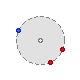FCSys.Species.Solid

Base model for an inert, stationary solid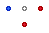### Information

Assumptions:

1. There is no material transport.
2. Velocity is zero.
3. There are no chemical reactions or phase change.
4. The volume is constant—determined by ε and the total volume of the subregion.

Extends from Species (Base model for one chemical species in one phase).

### Parameters

TypeNameDefaultDescription
Integern_inter0Number of exchange connections with other phases
Geometry
NumberAbsoluteepsilon0.25Volumetric fill fraction 
LengthkL[:]L[cartTrans]Effective transport length [L]
Material properties
replaceable package DataCharacteristics.BaseClasses….Characteristic data
MobilitymuData.mu(T, v)Mobility [N.T/M]
TimeAbsolutenuData.nu(T, v)Thermal independity [T]
Independence factors
NumberAbsolutek_intra_Phi[n_intra, n_trans]ones(n_intra, n_trans)For translational exchange among species within the phase 
NumberAbsolutek_intra_Q[n_intra]ones(n_intra)For thermal exchange among species within the phase 
Initialization
TemperatureAbsoluteT.startT_ICTemperature [L2.M/(N.T2)]
Velocityphi.start[n_trans]0Velocity [L/T]
Assumptions
Integern_trans1Number of transport axes
Formulation of the conservation equations
ConsThermoconsEnergyConsThermo.dynamicEnergy
Initialization
Densityrho_IC1/Data.v_Tp()Initial density [N/L3]
VolumeV_ICepsilon*product(L)Initial volume [L3]
PressureAbsolutep_ICenvironment.pInitial pressure [M/(L.T2)]
TemperatureAbsoluteT_IC Initial temperature [L2.M/(N.T2)]

### Connectors

TypeNameDescription
Intraintra[n_intra]Connectors to exchange translational momentum and energy within the phase
Interinter[n_inter]Connectors to exchange translational momentum and energy with all other species
ThermalDiffusiveboundaries[n_trans, Side]Connectors for transport

### Modelica definition

```model Solid "Base model for an inert, stationary solid"
import FCSys.Utilities.Delta;

// Geometry
parameter Q.NumberAbsolute epsilon=0.25 "Volumetric fill fraction";
extends Species(
final N_IC,
final V_IC=epsilon*product(L),
final rho_IC=1/Data.v_Tp(),
final p_IC=environment.p,
final h_IC,
final g_IC,
T(stateSelect=if consEnergy == ConsThermo.dynamic then StateSelect.always
else StateSelect.default,fixed=consEnergy == ConsThermo.dynamic));
parameter Q.Length kL[:]=L[cartTrans] "Effective transport length";
// Note:  The size is n_trans, but it isn't specified here to prevent a
// warning in Dymola 2014.

// Material properties
Q.ResistivityThermal theta(nominal=10*U.cm/U.A) = Data.theta(T, v)
"Thermal resistivity";

// Assumptions
parameter ConsThermo consEnergy=ConsThermo.dynamic "Energy";

// Auxiliary variables (for analysis)
output Q.TimeAbsolute tau_QT[n_trans](
each stateSelect=StateSelect.never,
each start=U.s) = N*c_v*theta*kL ./ (2*Aprime) if environment.analysis
"Time constants for thermal transport (through the whole subregion)";

output Q.Temperature DeltaT[n_trans](each stateSelect=StateSelect.never) =
Delta(boundaries.T) if environment.analysis
"Differences in temperatures across the boundaries";
Connectors.ThermalDiffusive boundaries[n_trans, Side](T(each start=T_IC))
"Connectors for transport";

protected
outer Q.Area Aprime[n_trans] "Effective cross-sectional area";

equation
// Assumptions
phi = zeros(n_trans) "Zero velocity";
V = epsilon*product(L) "Prescribed volume";

// Equation of state
if Data.isCompressible then
p = Data.p_Tv(T, v);
else
v = Data.v_Tp(T, p);
end if;

// Thermal transport (conduction)
for i in 1:n_trans loop
for side in Side loop
kL[i]*theta*boundaries[i, side].Qdot = 2*Aprime[i]*(boundaries[i, side].T -
T);
end for;
end for;

// Thermal dynamics
if consEnergy == ConsThermo.IC then
// Apply the IC forever (energy not conserved).
T = T_IC;
else
(if consEnergy == ConsThermo.dynamic then N*T*der(s)/U.s else 0) = sum(
intra[i].phi*intra[i].mPhidot for i in 1:n_intra) + sum(inter[i].phi*
inter[i].mPhidot for i in 1:n_inter) + sum(intra.Qdot) + sum(inter.Qdot) +
sum(boundaries.Qdot) "Conservation of energy";
end if;

end Solid;
```

##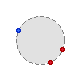FCSys.Species.Species

Base model for one chemical species in one phase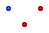### Information

All of the details below are pertinent to the Fluid model (and the derived Ion model) which inherits from this model. Only some of the details apply to the Solid model because it excludes the transport and exchange of material.

This model is based on the following fixed assumptions:

1. All boundaries are rectangular.
2. The material is orthorhombic. This implies that a gradient which induces diffusion along an axis does not induce diffusion along axes orthogonal to it [Bejan2006, pp. 691–692].
3. The coordinate system (x, y, z) is aligned with the principle axes of transport. For example, if the material is stratified, then the layers must be parallel to one of the planes in the rectilinear grid.
4. The effective transport lengths (kL) are common to material, translational, and thermal transport.
5. There is no radiative heat transfer (or it must be linearized and added to the thermal conductance).
6. Rotational momentum is not stored or transfered.
Other assumptions are optional via the parameters. Additional assumptions may be applied in models that inherit from this one.

Figure 1 shows how instances of Species models are connected within a Subregion. A single species in a single phase is called a configuration. The generalized resistances (R) affect the force and rates of chemical exchange and heat flow associated with differences in activity, velocity, and temperature (respectively) between each configuration and a common node. These exchange processes are diffusive. Each resistor generates heat in the Species instance that contains it.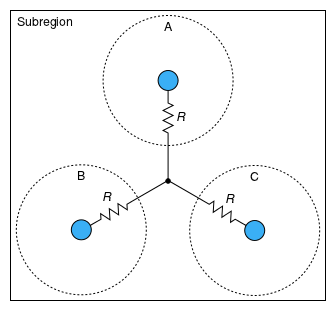Figure 1: Exchange of a quantity (material, translational momentum, or thermal energy) among configurations (A, B, and C) within a subregion.

Figure 2 shows how a configuration is connected between neighboring instances of a Subregion. Material, translational momentum, and thermal energy are transported by both advection and diffusion. Upstream discretization is applied if it is enabled via the `upstreamX`, etc. parameters. Like for exchange, the transport resistances are inside the Species model.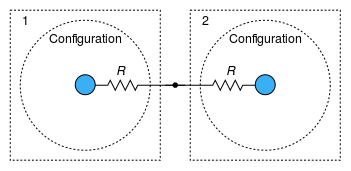Figure 2: Transport of a quantity associated with the same configuration between subregions (1 and 2).

The Species instances within a Phase are combined by Dalton's law of partial pressures (see the Dalton connector), as shown in Figure 3a. The pressures are additive, and each species is assumed to exist at the total extensive volume of the phase. Within a Subregion, the Phases are combined by Amagat's law of partial volumes (see the Amagat connector), as shown in Figure 3b. The volumes are additive, and each species is assumed to exist at the total pressure in the subregion.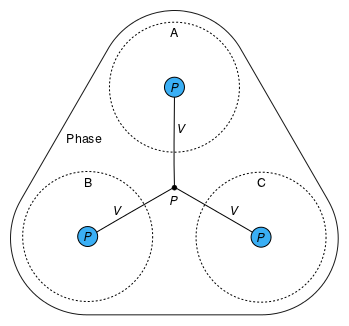a: Pressures of species (A, B, and C) are additive within a phase.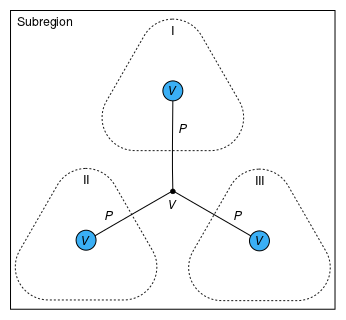b: Volumes of phases (I, II, and III) are additive within a subregion. Figure 3: Methods of attributing pressure and volume.

Notes regarding the parameters:

1. The effective transport lengths (kL) may be different that the geometric lengths along the transport axes due to tortouisity. The tortouisity may be anisotropic.
2. If the interval for chemical exchange (τ′), mobility (μ), thermal independity (ν), fluidity (η), or thermal resistivity (θ) is zero, then it should usually be set as `final` so that index reduction may be performed. If two configurations are connected through their `intra`, `inter`, or `boundaries` connectors and both have zero generalized resistivities for a quantity, then index reduction [Mattsson1993] is necessary.
3. Even if an initialization parameter is not selected for explicit use, it may be used a guess value.
4. If `ConsThermo.IC` is used for a state (via `consMaterial` or `consEnergy`), then the associated initial condition (IC) will be applied forever instead of the corresponding conservation equation.
5. If `consEnergy` is `ConsThermo.steady`, then NTs/∂t + Mφ∂φ/∂t is treated as zero and removed from the energy balance.
6. If a transport axis is not included (via the outer `inclTrans[:]` parameter which maps to `{inclTransX, inclTransY, inclTransZ}` in the Subregion model), then the associated pair of boundaries is removed from the model.
7. The `start` values of the initial conditions for pressure and temperature (pIC and TIC) are the global default pressure and temperature (via the `outer` instance of the Environment model). The `start` values of the initial conditions for other intensive properties (ρIC, hIC, and gIC) are related to the initial pressure and temperature by the characteristics of the species. The `start` value of the initial condition for the extensive volume (VIC) is the volume of the subregion. The `start` value for particle number (NIC) is related to it via the material characteristics and the initial pressure and temperature. In order to apply other values for any of these initial conditions, it may be necessary to do so before translating the model.
8. In the `boundaries` connector array, the transverse translational flow (mΦdot) is only the force due to diffusion. Translational advection is calculated from the current and the velocity. The thermal flow () is only the rate of heat transfer due to diffusion. The advection of thermal energy is determined from the current and the thermodynamic state at the boundary.

For the variables that relate to transport, the first index is the axis and the second index is the side. The sides are ordered from negative to positive, according to the Side enumeration. Velocity and force are additionally indexed by the orientation of the momentum with respect to the boundary. The orientations are ordered following the normal axis in Cartesian space, according to the Orient enumeration.

### Parameters

TypeNameDefaultDescription
Integern_inter0Number of exchange connections with other phases
Material properties
replaceable package DataCharacteristics.BaseClasses….Characteristic data
MobilitymuData.mu(T, v)Mobility [N.T/M]
TimeAbsolutenuData.nu(T, v)Thermal independity [T]
Independence factors
NumberAbsolutek_intra_Phi[n_intra, n_trans]ones(n_intra, n_trans)For translational exchange among species within the phase 
NumberAbsolutek_intra_Q[n_intra]ones(n_intra)For thermal exchange among species within the phase 
Assumptions
Integern_trans1Number of transport axes
Initialization
AmountN_IC Initial amount of material [N]
Densityrho_IC Initial density [N/L3]
VolumeV_IC Initial volume [L3]
PressureAbsolutep_IC Initial pressure [M/(L.T2)]
TemperatureAbsoluteT_IC Initial temperature [L2.M/(N.T2)]
Potentialh_IC Initial specific enthalpy [L2.M/(N.T2)]
Potentialg_IC Initial Gibbs potential [L2.M/(N.T2)]

### Connectors

TypeNameDescription
Intraintra[n_intra]Connectors to exchange translational momentum and energy within the phase
Interinter[n_inter]Connectors to exchange translational momentum and energy with all other species
Material properties
replaceable package DataCharacteristic data

### Modelica definition

```partial model Species
"Base model for one chemical species in one phase"

import assert = FCSys.Utilities.assertEval;

// Material properties
replaceable package Data = Characteristics.BaseClasses.Characteristic
constrainedby Characteristics.BaseClasses.Characteristic
"Characteristic data";
Q.Mobility mu(nominal=10*U.cm^2/(U.V*U.s)) = Data.mu(T, v) "Mobility";
Q.TimeAbsolute nu(nominal=1e-9*U.s) = Data.nu(T, v) "Thermal independity";

// Assumptions
parameter Integer n_trans=1 "Number of transport axes";
// This can't be an outer parameter in Dymola 2014.
parameter Integer n_intra=0 "Number of exchange connections within the phase";
parameter Integer n_inter=0
"Number of exchange connections with other phases";

// Initialization parameters
parameter Q.Amount N_IC(start=V_IC*rho_IC) "Initial amount of material";
parameter Q.Density rho_IC(start=1/Data.v_Tp(T_IC, p_IC)) "Initial density";
parameter Q.Volume V_IC(start=product(L)) "Initial volume";
parameter Q.PressureAbsolute p_IC(start=environment.p) "Initial pressure";
parameter Q.TemperatureAbsolute T_IC(start=environment.T)
"Initial temperature";
parameter Q.Potential h_IC(start=Data.h(T_IC, p_IC), displayUnit="kJ/mol")
"Initial specific enthalpy";
parameter Q.Potential g_IC(start=Data.g(T_IC, p_IC), displayUnit="kJ/mol")
"Initial Gibbs potential";

// Independence factors
parameter Q.NumberAbsolute k_intra_Phi[n_intra, n_trans]=ones(n_intra,
n_trans) "For translational exchange among species within the phase";
parameter Q.NumberAbsolute k_intra_Q[n_intra]=ones(n_intra)
"For thermal exchange among species within the phase";

// Preferred states
// Note:  The start values for these variable aren't fixed because the
// initial equation section will be used instead.
Q.Amount N(
final min=Modelica.Constants.small,
nominal=4*U.C,
final start=N_IC,
final fixed=false,
stateSelect=StateSelect.prefer) "Amount of material";
Q.TemperatureAbsolute T(
nominal=300*U.K,
final start=T_IC,
stateSelect=StateSelect.prefer) "Temperature";
Q.Velocity phi[n_trans](
each nominal=100*U.cm/U.s,
each start=0,
each stateSelect=StateSelect.prefer) "Velocity";

// Aliases (for common terms)
// Note:  StateSelect.never helps avoid dynamic state selection of these
// variables in Dymola 2014.
Q.PressureAbsolute p(
nominal=U.atm,
final start=p_IC,
final fixed=false,
stateSelect=StateSelect.never) "Pressure";
Q.Potential g(
nominal=U.V,
final start=g_IC,
final fixed=false,
stateSelect=StateSelect.never) "Specific Gibbs energy";
Q.Mass M(
nominal=1e-3*U.g,
final start=Data.m*N_IC,
stateSelect=StateSelect.never) "Mass";
Q.VolumeSpecific v(
nominal=U.cc/(4*U.C),
final start=1/rho_IC,
final fixed=false,
stateSelect=StateSelect.never) "Specific volume";
Q.Potential h(
nominal=U.V,
final start=h_IC,
final fixed=false,
stateSelect=StateSelect.never) "Specific enthalpy";
Q.NumberAbsolute s(
nominal=25,
final start=(h_IC - g_IC)/T_IC,
stateSelect=StateSelect.never) "Specific entropy";

// Auxiliary variables (for analysis)
// ----------------------------------
// Thermodynamic properties
output Q.Density rho(stateSelect=StateSelect.never) = 1/v if environment.analysis
"Density";
output Q.MassVolumic mrho(stateSelect=StateSelect.never) = Data.m*rho if
environment.analysis "Volumic mass";
output Q.Amount S(stateSelect=StateSelect.never) = N*s if environment.analysis
"Entropy";
output Q.CapacityThermalSpecific c_p(stateSelect=StateSelect.never) =
Data.c_p(T, p) if environment.analysis "Isobaric specific heat capacity";
output Q.CapacityThermalSpecific c_v(stateSelect=StateSelect.never) =
Data.c_v(T, p) if environment.analysis "Isochoric specific heat capacity";
output Q.PressureReciprocal beta(stateSelect=StateSelect.never) = Data.beta(T,
p) if environment.analysis "Isothermal compressibility";
//
// Time constants
output Q.TimeAbsolute tau_PhiE_intra[n_intra, n_trans](
each stateSelect=StateSelect.never,
each start=U.s) = {Data.m*mu*k_intra_Phi[i, :] for i in 1:n_intra} if
environment.analysis and n_intra > 0
"Time constants for translational intra-phase exchange";
output Q.TimeAbsolute tau_PhiE_inter[n_inter, n_trans](
each stateSelect=StateSelect.never,
each start=U.s) = {Data.m*mu*k_inter_Phi[i, :] for i in 1:n_inter} if
environment.analysis and n_inter > 0
"Time constants for translational inter-phase exchange";
output Q.TimeAbsolute tau_QE_intra[n_intra](
each stateSelect=StateSelect.never,
each start=U.s) = c_p*nu*k_intra_Q if environment.analysis and n_intra > 0
"Time constants for thermal intra-phase exchange";
output Q.TimeAbsolute tau_QE_inter[n_inter](
each stateSelect=StateSelect.never,
each start=U.s) = c_p*nu*k_inter_Q if environment.analysis and n_inter > 0
"Time constants for thermal inter-phase exchange";
// Note:  The structure of the problem should not change if these auxiliary
// variables are included (hence, StateSelect.never).
//
// Translational momentum balance
output Q.Force f_DE[n_trans](each stateSelect=StateSelect.never) = sum(intra[
i].mPhidot for i in 1:n_intra) + sum(inter[i].mPhidot for i in 1:n_inter)
if environment.analysis
"Friction from other configurations (diffusive exchange)";
//
// Energy balance
output Q.Power Edot_DE(stateSelect=StateSelect.never) = sum(intra[i].phi*
intra[i].mPhidot for i in 1:n_intra) + sum(inter[i].phi*inter[i].mPhidot
for i in 1:n_inter) + sum(intra.Qdot) + sum(inter.Qdot) if environment.analysis
"Rate of diffusion of energy from other configurations";
Connectors.Intra intra[n_intra](each final n_trans=n_trans, each T(final
start=T_IC, final fixed=false))
"Connectors to exchange translational momentum and energy within the phase";
Connectors.Inter inter[n_inter](each final n_trans=n_trans, each T(final
start=T_IC, final fixed=false))
"Connectors to exchange translational momentum and energy with all other species";

Connectors.Dalton dalton(V(
min=0,
final start=V_IC,
final fixed=false), p(final start=p_IC, final fixed=false))

// Geometric parameters

protected
outer Q.Volume V "Volume of the phase (not of the subregion)";
outer parameter Q.Length L[Axis] "Lengths of the subregion";
outer parameter Integer cartTrans[:]
"Cartesian-axis indices of the transport axes";
// Note:  The size of cartTrans is n_trans, but it can't be specified here
// due to an error in Dymola 2014.
outer parameter Q.NumberAbsolute k_inter_Phi[:, :]
"Independence factors for translational exchange with other phases";
outer parameter Q.NumberAbsolute k_inter_Q[:]
"Independence factors for thermal exchange among with other phases";
// Note:  The sizes can't be specified due to an error in Dymola 2014.

outer Conditions.Environment environment "Environmental conditions";

initial equation
// Check the initial conditions.
assert(V >= 0, "The volume of " + getInstanceName() + " is negative.
Check that the volumes of the other phases are set properly.");

equation
// Aliases (only to clarify and simplify other equations)
h = g + T*s;
p = dalton.p;
v*N = dalton.V;
M = Data.m*N;

// Thermodynamic correlations
h = Data.h(T, p);
s = Data.s(T, p);

// Exchange
// --------
// Within the phase
for i in 1:n_intra loop
k_intra_Phi[i, :]*mu .* intra[i].mPhidot = N*(intra[i].phi - phi)
"Translational";
k_intra_Q[i]*nu*intra[i].Qdot = N*(intra[i].T - T) "Thermal";
end for;
//
// With other phases
for i in 1:n_inter loop
k_inter_Phi[i, :]*mu .* inter[i].mPhidot = N*(inter[i].phi - phi)
"Translational";
k_inter_Q[i]*nu*inter[i].Qdot = N*(inter[i].T - T) "Thermal";
end for;

end Species;
```